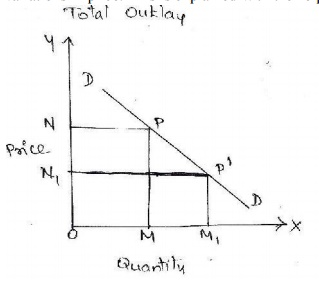Home | Different Methods of Measuring Elasticity of Demand

# Different Methods of Measuring Elasticity of Demand

1. Total outlay 2. Point elasticity 3. Arc elasticity

DIFFERENT METHODS OF MEASURING ELASTICITY OF DEMAND

1. Total outlay: According to this method, we compare the total outla y of the purchases or total revenue, i.e, total value o f sales from the po int of view of the seller before and after the variations in price. This is explained with the help of a diagram.X axis -------Quantity of X

Y axis---------price of X

DD ------------demand curve

Explanation: If the elasticity of demand is equal to unity for all prices of the commodity only fall in price will cause a proportionate increases in the amount bought, and therefore will make no change in the total outla y which purchases make for the commodity thus one is the dividing point. If the elasticity is greater than one it is said to be elast ic and it is less than it is inelastic curve having same elasticity throughout:-

2. Point elasticity: The concept of price elasticity can be used in comparing the sensitivity of the different types of goods e.g., luxuries and necessaries) to changes in their prices. The elasticity of d emand is alwa ys negative because change in quantity demanded is in opposite direction to the change in price that is a fall in p rice is followed b y rise in demanded and vice versa hence elasticity less than zero.X axis -------Quantity of X

Y axis---------price of X

DD ------------demand curve

Explanation:

Elasticity is represented b y fraction distance fro m d to a point on the curve divided b y the distance from the other end to that point. Thus elasticity of demand is seen on the points P3, P2and P1 respectively. It is seen that elasticity at a lower point on the curve is less than at a higher point.

3. Arc elasticity: Arc elasticity is a measure of the average responsiveness to price changes exhibited by a demand curve over some finite stretch of the curve. This is explained with the help of a diagram below.X axis -------Quantity of X

Y axis---------price of X

DD ------------demand curve

Explanation:-

i. Any two points on a demand curve make an arc the area between p and m on the DD curve is

an arc which measures elasticity over a certain range of prices and quantities.

different depending upon how we calculate them.

iii. The closer thetwopoints'pandmare,themoreaccuratewilbethemeasureofelasticity. iv. The arc elasticity is in fact the elasticity of the midpoint between p and m on the demand

curve .

v. If there is no difference between the two points and the y merge into each o ther o r coincide, arc elasticity becomes point elasticity.

Study Material, Lecturing Notes, Assignment, Reference, Wiki description explanation, brief detail
Civil : Engineering Economics and Cost analysis : Demand and Schedule : Different Methods of Measuring Elasticity of Demand |